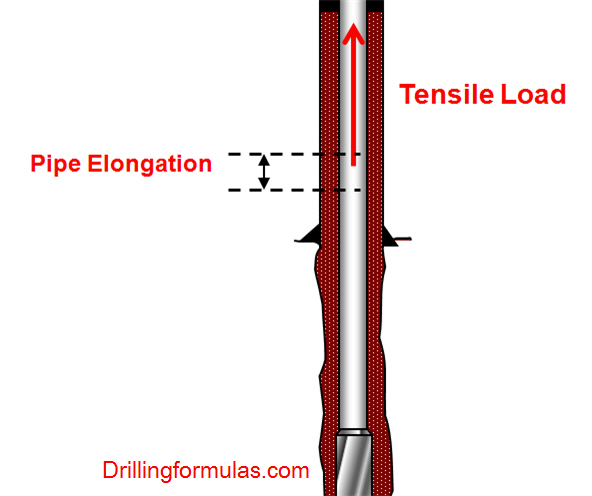# Drillstring Elongation Due To a Tensile Load

When a tensile load is applied into a drillstring, it results in elongation of the drillstring.The formula to determine pipe elongation is listed below;

Oilfield unit

e = (L x T) ÷ (735,000 x W)

Where;

e = elongation, inch

L = length of drill string, ft

W = drill string weight, lb/ft

Metric (SI) unit

e = (L x T) ÷ (26.7 x W)

Where;

e = elongation, mm

L = length of drill string, metres

W = drill string weight, kg/m

Note: The equations are taken from API RP 7G 16th Edition, August 1998.

Determine the pipe elongation from the following data (this example will use “oilfield unit” for calculation.

5” DP, S-135, 4-1/2” IF Connection. The pipe specification is listed below.W = 23.52 ppf

Length = 8,500 ft

Pick up weight = 300 Klb

Block weight without drill string = 70 Klb

Tensile load applied into the drill string is equal to pick up weight minus block weight.

T = 300 – 70 = 230 Klb

e = (8,500 x 230,00) ÷ (735,000 x 23.52)

e = 113.1  inch

Ref books:Drilling engineering books

Share the joyWorking in the oil field and loving to share knowledge.

### 4 Responses to Drillstring Elongation Due To a Tensile Load

1.SAMUEL ANIGBORO says:

Good stuff for oil field duds.

2.Ramiro Rivera C. says:

Very usefull

3.rohit bhosale says:

How did you derive the formula to determine the drill pipe elongation due to tension?

•DrillingFormulas.Com says:

This is derived from Young’s Modulus relationship.

This site uses Akismet to reduce spam. Learn how your comment data is processed.